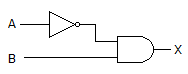# Digital Electronics - Logic Gates

### Exercise :: Logic Gates - Filling the Blanks

1.

The gates in this figure are implemented using TTL logic. If the input of the inverter is open, and you apply logic pulses to point B, the output of the AND gate will be ________.A. a steady LOW B. a steady HIGH C. an undefined level D. pulses

Explanation:

No answer description available for this question. Let us discuss.

2.

The gates in this figure are implemented using TTL logic. If the output of the inverter is open, and you apply logic pulses to point B, the output of the AND gate will be ________.A. a steady LOW B. a steady HIGH C. an undefined level D. pulses

Explanation:

No answer description available for this question. Let us discuss.

3.

If A is LOW or B is LOW or BOTH are LOW, then X is LOW. If A is HIGH and B is HIGH, then X is HIGH. These rules specify the operation of a(n) ________.

 A. AND gate B. OR gate C. NAND gate D. XOR gate

Explanation:

No answer description available for this question. Let us discuss.

4.

A major advantage of ECL logic over TTL and CMOS is ________.

 A. low power dissipation B. high speed C. both low power dissipation and high speed D. neither low power dissipation nor high speed

Explanation:

No answer description available for this question. Let us discuss.

5.

The output of an XOR gate is HIGH only when ________.

 A. both inputs = 0 B. both inputs = 1 C. the two inputs are unequal D. both inputs are undefined